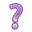## 8.5 Binary predictors.

Does gender also play a roll in FEV? Let’s look at the separate effects of height and age on FEV1, and visualize how gender plays a roll.

ht.plot <- ggplot(fev_long, aes(x=ht, y=fev1)) +
geom_point(aes(col=gender)) +
geom_smooth(se=FALSE, aes(col=gender), method="lm") +
geom_smooth(se=FALSE, col="red", method="lm") +
scale_color_viridis_d() +
theme(legend.position = c(0.15,0.85))

age.plot <- ggplot(fev_long, aes(x=age, y=fev1)) +
geom_point(aes(col=gender)) +
geom_smooth(se=FALSE, aes(col=gender), method="lm") +
geom_smooth(se=FALSE, col="red", method="lm") +
scale_color_viridis_d(guide=FALSE)

grid.arrange(ht.plot, age.plot, ncol=2)• The points are colored by gender
• Each gender has it’s own best fit line in the same color as the points
• The red line is the best fit line overall - ignoring genderIs gender a moderator for either height or age?

Let’s compare the models with, and without gender

 Dependent variable: fev1 W/o gender w/ gender age -0.02*** (-0.03, -0.01) -0.02*** (-0.03, -0.02) ht 0.16*** (0.15, 0.18) 0.11*** (0.08, 0.13) genderF -0.64*** (-0.79, -0.48) Constant -6.74*** (-7.84, -5.63) -2.24*** (-3.71, -0.77) Observations 300 300 Adjusted R2 0.57 0.65 Residual Std. Error 0.53 (df = 297) 0.48 (df = 296) F Statistic 197.57*** (df = 2; 297) 182.77*** (df = 3; 296) Note: p<0.1; p<0.05; p<0.01
• Gender is a binary categorical variable, with reference group “Male”.
• This is detected because the variable that shows up in the regression model output is genderF. So the estimate shown is for males, compared to females.
• More details on how categorical variables are included in multivariable models is covered in section 8.6.

Interpretation of Coefficients

The regression equation for the model without gender is

$y = -6.74 - 0.02 age + 0.16 height$

• $$b_{0}:$$ For someone who is 0 years old and 0 cm tall, their FEV is -6.74L.
• $$b_{1}:$$ For every additional year older an individual is, their FEV1 decreases by 0.02L.
• $$b_{2}:$$ For every additional cm taller an individual is, their FEV1 increases by 0.16L.

The regression equation for the model with gender is

$y = -2.24 - 0.02 age + 0.11 height - 0.64genderF$

• $$b_{0}:$$ For a male who is 0 years old and 0 cm tall, their FEV is -2.24L.
• $$b_{1}:$$ For every additional year older an individual is, their FEV1 decreases by 0.02L.
• $$b_{2}:$$ For every additional cm taller an individual is, their FEV1 increases by 0.16L.
• $$b_{3}:$$ Females have 0.64L lower FEV compared to males.

Note: The interpretation of categorical variables still falls under the template language of “for every one unit increase in $$X_{p}$$, $$Y$$ changes by $$b_{p}$$”. Here, $$X_{3}=0$$ for males, and 1 for females. So a 1 “unit” change is females compared to males.Which model fits better? What measure are you using to quanitify “fit”?

What part of the model (intercept, or one of the slope parameters) did adding gender have the most effect on?